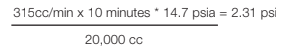# Integrity Testing: Pressure Hold

Integrity testing is considered essential in those processes that utilize a membrane filter for bioburden control to assure that the filter meets the removal criteria. Bubble point and diffusive flow (forward flow) are the most common methods. However, in instances where a multi-round housing is utilized, it becomes impossible to distinguish diffusive flow from the flow of a failed device, as the volume of air passing may be quite significant. In these instances, the Pressure Hold Test, also known as Pressure Decay Test, is recommended. However, unlike the bubble point or diffusive flow, the Pressure Hold value is not published since it is unique to each system and must be calculated based upon system volume and time.

Pressure hold test is a non-destructive integrity test based upon the diffusive flow (forward flow) of a cartridge. Using a highly accurate gauge, the upstream pressure change due to gas diffusion through the filter in monitored over time. Because the measurement is upstream of the filters, any risk to downstream sterility is eliminated. The value is highly dependent upon the volume of the system as any loss of pressure would be due to the flow that diffuses through the cartridge (a wetted cartridge is impermeable to bulk flow of air, but diffusion occurs once positive pressure is applied upstream). By knowing the maximum diffusive flow of a cartridge and the volume of the system (the larger the system, the less the pressure drop would be), it is possible to calculate the maximum permissible pressure drop based upon the following equation:

Pressure Hold Test Formula:
D (T) (Pa)/ Vh = ÄP

Where:
D = Diffusion rate (cc/min)
T = Time (minutes)
Pa = Atmosphere pressure (1 Atm. or 14.7 psi)
Vh = Upstream volume of apparatus (cc)
ÄP = Pressure Drop (bar or psi)

Example:
The ZTEC WB 0.45 micron has a maximum diffusive flow
value of 35 cc/min at 16 psig. Therefore a 30” cartridge
would have a maximum value of 105 cc/min (35x3) at
16 psig. Three cartridges would therefore have a value of
315 cc/min (105x3) at 16 psig.In this case, if the pressure drop in the system is less than 2.31 psi during the 10 minute test, the system would be considered integral. Since volume is a critical part of the calculation, an accurate value must be determined for the system being tested.

The system volume would include the housing volume as well as the volume of the piping/tubing from the inlet valve and upstream gas valve. Due to this potential volume variation, this value is not provided by the cartridge filter manufacturer, but rather must be calculated or empirically derive. The calculation should include the housing volume (which is a value the housing manufacturer can provide) as well all piping/tubing between the valves that are used as part of the sealed system. Alternatively, the volume of the system can be empirically derived by actually measuring the volume by filling the system with water and then measuring the volume added.

Updated

3 out of 3 found this helpful

Have more questions? Submit a request

<% if (previousArticle || nextArticle) { %>
<% if (previousArticle) { %>
<% if (previousTitle) { %>

#### <%= previousTitle %>

<% } %> <%= previousArticle.title %>
<% } %> <% if (nextArticle) { %>
<% if (nextTitle) { %>

#### <%= nextTitle %>

<% } %> <%= nextArticle.title %>
<% } %>
<% } %>
<% if (allItems.length > 1) { %>